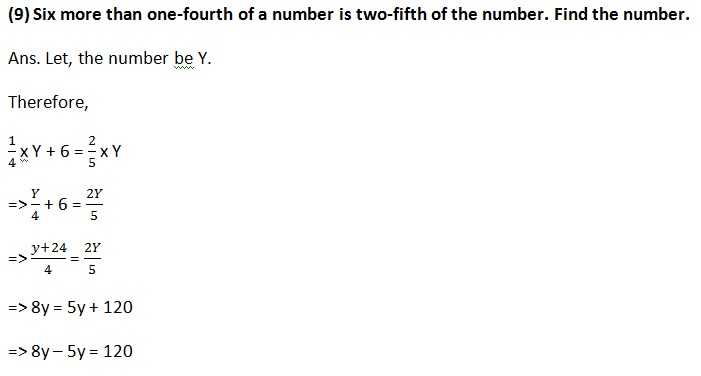# Selina Concise Class 8 Math Chapter 14 Linear Equations in One Variable Exercise 14B Solution

Concise Mathematics – Class 8 Selina Exercise 14B Solution by experts | 14B Selina Concise Solution Class 8 | Class 8 Selina Concise Ex 14B Page 169 Solution | RK Bansal Class 8 ICSE Book Solution Online.

### Exercise 14(B)

(1) Fifteen less than 4 times a number is 9. Find the number.

Solution: Let the number be x

Therefore, 4x – 15 = 9

=> 4x = 9 + 15

=> 4x = 24

=> x = 24 / 4

=> x = 6

(2) If Megha’s age is increased by three times her age, the result is 60 years. Find her age.

Solution: Let Megha’s age be x years.

Therefore, x + 3x = 60

=> 4x = 60

=> x = 60 / 4

=> x = 15

Ans. Her age is 15 years

(3) 28 is 12 less than 4 times a number. Find the number.

Solution: Let the number be x

Therefore,

28 = 4x – 12

=> 4x – 12 = 28

=> 4x = 28 + 12

=> 4x = 40

=> x = 40 / 4

=> x = 10

(4) Five less than 3 times a number is – 20. Find the number.

Solution: Let the number be x

Therefore,

3x – 5 = -20

=> 3x = -20 + 5

=> 3x = -15

=> x = -5

Ans. The number is -5.

(5) Fifteen more than 3 times Neetu’s age is the same as 4 times her age. How old is she?

Solution: Let Neetu’s age be x years.

Therefore,

3x + 15 = 4x

=> 3x – 4x = – 15

=> -1x = -15

=> x = 15

Ans Her age is 15 years.

(6) A number decreased by 30 is the same as 14 decreased by 3 times the number. Find the number.

Solution: Let the number be x

Therefore,

X – 30 = 3x – 14

=> x + 3x = – 14 + 30

=> 4x = 44

=> x = 11

The number is 11

(7) A’s salary is same as 4 times  B’s salary. If together they earn Rs. 3,750 a month, find the salary of each.

Ans. Let A’s salary be x

B’s salary will 4x

Therefore,

X + 4x = 3750

=> 5x = 3750

=> x = 3750 / 5

=> x = 750

A’s salary is Rs. 750

B’s salary is 4 x 750 = Rs. 3000

(8) Separate 178 in two parts so that the first part is 8 less than twice the second part.

Ans. Let the second part be ‘x’

Then the first part is 2x – 8

By the problem, x+2x-8=178

3x-8=178

3x=178+8=186

x=186/3 = 62

So the first part is 2x-8 = 2(62)-8 = 124-8=116

Second part is x = 62=> 3y = 120

=> y = 120/3

=> y = 40

The number is 40

(10) Let the width be x.

Length  = 2x – 5

Therefore,

2 x (L + B) = Perimiter

=> 2 x (2x – 5 – 5 + x + 2) = 74

=> 2 x (3x – 8) = 74

=> 6x – 16 = 74

=> 6x = 74 + 16

=> 6x = 90

=> x = 90 / 6

=> x = 15

The length of the original rectangle is 2 x 15 – 5 = 30 – 5 = 25 cm

Width is 15 cm

(12) Let the three consecutive odd number be x, (x + 2) and (x |+ 4)

Therefore,

X + (x + 2) + (x + 4) = 57

=> 3x + 6 = 57

=> 3x = 57 – 6

=> 3x = 51

=> x = 17

The three numbers are 17, 17 + 2 = 19, 17 + 4 = 21

(13) Let sons present age be x

Man’s age = 3x

In 12 years Sons age become = x + 12

In 12 years Mans age become = 3x + 12

Therefore,

3x + 12 = 2(x+12)

=> 3x + 12 = 2x + 24

=> 3x – 2x = 24 – 12

=> 1x = 12

=> x = 12

Son’s present age  = 12 years

Mans present age = 3 x 12 = 36 years.

(14) Let in x years will the age will the age of the son be half the age of the man the age of the man at that time.

After x years Man’s age = 42 + x

After x years Son’s age = 12 + x

Therefore,

42 + x = 2(12+x)

=> 42 + x = 24 + 2x

=> x – 2x = 24 – 42

=> -1x = -18

=> x = 18

Ans. 18 years.=> 5x+816-6x = 720

=> -1x = 720 – 816

=> -1x = -96

=> x = 96

Ans. 96 km covered at 18 km/hr.

(16) Let the first number be x

And the second number be x + 3

Therefore,

(x+3)2 – x2 = 69

=> x2 + 6x + 9 – x2 = 69

=> 6x = 69 – 9

=> 6x = 60

=> x = 10

The first number is 10

And the second number is = 10 + 3 = 13

(17) Let, the two consecutive number x and x+1

Therefore(20) Let, present age of sons be x

Mans age = 2x

Eight years hence Mans age be 2x + 8

Son’s age be = x + 8

Therefore,=> 8x + 32 = 7x + 56

=> 8x – 7x = 56 – 32

=> x = 24

The present age of man and son are = 2×24 = 48 years and 24 years respectively.

Updated: August 16, 2019 — 2:04 pm